7835 Solartron Liquid Densitometer

Uncorrected Observed Density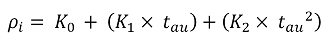Symbol Description Units
ρi Uncorrected observed density kg/m3
K0 Obtained from the calibration certificate -
K1 Obtained from the calibration certificate -
K2 Obtained from the calibration certificate -
tau Densitometer period µs

Correction for Temperature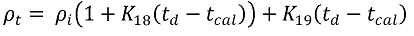Symbol Description Units
ρt Temperature corrected observed density kg/m3
ρi Uncorrected observed density kg/m3
K18 Obtained from the calibration certificate -
K19 Obtained from the calibration certificate -
td Temperature at the densitometer °C
tcal Densitometer calibrated temperature °C

Default Method for K20 and K21 Calculation

The method used if the DECC update is NOT checked in the configuration options: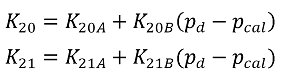Symbol Description Units
K20 Used to calculate pressure corrected observed density -
K21 Used to calculate pressure corrected observed density -
K20A Obtained from the calibration certificate -
K20B Obtained from the calibration certificate -
K21A Obtained from the calibration certificate -
K21B Obtained from the calibration certificate -
pd Pressure at the densitometer bar g
pcal Densitometer calibrated pressure bar g

The DECC Policy Statement on Traceable Density Measurement (30/09/2010) announced that Micro Motion would be releasing new density constants and equations that are optimised to be valid over the full operating calibration range of each densitometer. If the configuration option 'Use DECC Update' is checked inputs for the additional two calibration constants K20C and K21C can be entered and the method below is used to calculate K20 and K21.

ρtp is still calculated using the same equation.

DECC Method for K20 and K21 Calculation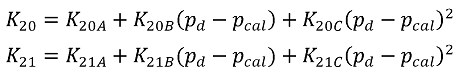Symbol Description Units
K20 Used to calculate pressure corrected observed density -
K21 Used to calculate pressure corrected observed density -
K20A Obtained from the calibration certificate -
K20B Obtained from the calibration certificate -
K20C Obtained from the calibration certificate -
K21A Obtained from the calibration certificate -
K21B Obtained from the calibration certificate -
K21C Obtained from the calibration certificate -
pd Pressure at the densitometer bar g
pcal Densitometer calibrated pressure bar g

Correction for Pressure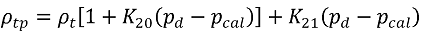Symbol Description Units
ρtp Pressure and temperature corrected observed density kg/m3
ρt Temperature corrected observed density kg/m3
K20 Obtained from the calibration certificate -
K21 Obtained from the calibration certificate -
pd Pressure at the densitometer bar g
pcal Densitometer calibrated pressure bar g

An additional correction is carried out when the 'Use DECC Update' is checked in the configuration options. Two extra inputs are needed, K22 and K23, to calculate the coupled observed density. These will be enterable when the DECC option is checked.

Temperature and Pressure Coupled Corrected Observed Density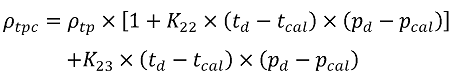Symbol Description Units
ρtpc Temperature and pressure coupled corrected observed density kg/m3
ρtp Pressure and temperature corrected observed density kg/m3
K22 Obtained from the calibration certificate -
K23 Obtained from the calibration certificate -
td Temperature at the densitometer °C
tcal Densitometer calibrated temperature °C
pd Pressure at the densitometer bar g
pcal Densitometer calibrated pressure bar g

References

• Solarton 7835/7845/7846/7847 Liquid Density Meter Technical Manual, Document Number - 78355080, 2008